► Welcome to mpost-wrapper.
 1 ex-1 1.1 source code in ex-1.rkt 1.2 the result of ex-1 2 ex-2 2.1 source code in ex-2.rkt 2.2 the result of ex-2 3 ex-3 3.1 source code in ex-3.rkt 3.2 the result of ex-3 4 ex-4 4.1 source code in ex-4.rkt 4.2 the result of ex-4 5 ex-5 5.1 source code in ex-5.rkt 5.2 the result of ex-5 6 ex-6 6.1 source code in ex-6.rkt 6.2 the result of ex-6 7 ex-7 7.1 source code in ex-7.rkt 7.2 the result of ex-7 8 ex-8 8.1 source code in ex-8.rkt 8.2 the result of ex-8 9 ex-9 9.1 source code in ex-9.rkt 9.2 the result of ex-9 10 ex-10 10.1 source code in ex-10.rkt 10.2 the result of ex-10 11 ex-11 11.1 source code in ex-11.rkt 11.2 the result of ex-11 12 ex-12 12.1 source code in ex-12.rkt 12.2 the result of ex-12 13 ex-13 13.1 source code in ex-13.rkt 13.2 the result of ex-13 14 ex-14 14.1 source code in ex-14.rkt 14.2 the result of ex-14 15 ex-15 15.1 source code in ex-15.rkt 15.2 the result of ex-15 16 ex-16 16.1 source code in ex-16.rkt 16.2 the result of ex-16 17 ex-17 17.1 source code in ex-17.rkt 17.2 the result of ex-17 18 ex-18 18.1 source code in ex-18.rkt 18.2 the result of ex-18 19 ex-19 19.1 source code in ex-19.rkt 19.2 the result of ex-19 20 ex-20 20.1 source code in ex-20.rkt 20.2 the result of ex-20 21 ex-21 21.1 source code in ex-21.rkt 21.2 the result of ex-21 22 ex-22 22.1 source code in ex-22.rkt 22.2 the result of ex-22 23 ex-23 23.1 source code in ex-23.rkt 23.2 the result of ex-23 24 ex-24 24.1 source code in ex-24.rkt 24.2 the result of ex-24 25 ex-25 25.1 source code in ex-25.rkt 25.2 the result of ex-25 26 ex-26 26.1 source code in ex-26.rkt 26.2 the result of ex-26 27 ex-27 27.1 source code in ex-27.rkt 27.2 the result of ex-27 28 ex-28 28.1 source code in ex-28.rkt 28.2 the result of ex-28 29 ex-29 29.1 source code in ex-29.rkt 29.2 the result of ex-29 30 ex-30 30.1 source code in ex-30.rkt 30.2 the result of ex-30 31 ex-31 31.1 source code in ex-31.rkt 31.2 the result of ex-31 32 ex-32 32.1 source code in ex-32.rkt 32.2 the result of ex-32 33 ex-33 33.1 source code in ex-33.rkt 33.2 the result of ex-33 34 ex-34 34.1 source code in ex-34.rkt 34.2 the result of ex-34 35 ex-35 35.1 source code in ex-35.rkt 35.2 the result of ex-35 36 ex-36 36.1 source code in ex-36.rkt 36.2 the result of ex-36 37 ex-37 37.1 source code in ex-37.rkt 37.2 the result of ex-37 38 ex-38 38.1 source code in ex-38.rkt 38.2 the result of ex-38 39 ex-39 39.1 source code in ex-39.rkt 39.2 the result of ex-39 40 ex-40 40.1 source code in ex-40.rkt 40.2 the result of ex-40 41 ex-41 41.1 source code in ex-41.rkt 41.2 the result of ex-41 42 ex-42 42.1 source code in ex-42.rkt 42.2 the result of ex-42 43 ex-43 43.1 source code in ex-43.rkt 43.2 the result of ex-43 44 ex-44 44.1 source code in ex-44.rkt 44.2 the result of ex-44 45 ex-45 45.1 source code in ex-45.rkt 45.2 the result of ex-45 46 ex-46 46.1 source code in ex-46.rkt 46.2 the result of ex-46 47 ex-47 47.1 source code in ex-47.rkt 47.2 the result of ex-47 48 ex-48 48.1 source code in ex-48.rkt 48.2 the result of ex-48 49 ex-49 49.1 source code in ex-49.rkt 49.2 the result of ex-49 50 ex-50 50.1 source code in ex-50.rkt 50.2 the result of ex-50 51 ex-51 51.1 source code in ex-51.rkt 51.2 the result of ex-51 52 ex-52 52.1 source code in ex-52.rkt 52.2 the result of ex-52 53 ex-53 53.1 source code in ex-53.rkt 53.2 the result of ex-53 54 ex-54 54.1 source code in ex-54.rkt 54.2 the result of ex-54 55 ex-55 55.1 source code in ex-55.rkt 55.2 the result of ex-55 56 ex-56 56.1 source code in ex-56.rkt 56.2 the result of ex-56 57 ex-57 57.1 source code in ex-57.rkt 57.2 the result of ex-57 58 ex-58 58.1 source code in ex-58.rkt 58.2 the result of ex-58 59 ex-59 59.1 source code in ex-59.rkt 59.2 the result of ex-59 60 ex-60 60.1 source code in ex-60.rkt 60.2 the result of ex-60 61 ex-61 61.1 source code in ex-61.rkt 61.2 the result of ex-61 62 ex-62 62.1 source code in ex-62.rkt 62.2 the result of ex-62 63 ex-63 63.1 source code in ex-63.rkt 63.2 the result of ex-63 64 ex-64 64.1 source code in ex-64.rkt 64.2 the result of ex-64 65 ex-65 65.1 source code in ex-65.rkt 65.2 the result of ex-65 66 ex-66 66.1 source code in ex-66.rkt 66.2 the result of ex-66 67 ex-67 67.1 source code in ex-67.rkt 67.2 the result of ex-67 68 ex-68 68.1 source code in ex-68.rkt 68.2 the result of ex-68 69 ex-69 69.1 source code in ex-69.rkt 69.2 the result of ex-69 70 ex-70 70.1 source code in ex-70.rkt 70.2 the result of ex-70 71 ex-71 71.1 source code in ex-71.rkt 71.2 the result of ex-71 72 ex-72 72.1 source code in ex-72.rkt 72.2 the result of ex-72 73 ex-73 73.1 source code in ex-73.rkt 73.2 the result of ex-73 74 ex-74 74.1 source code in ex-74.rkt 74.2 the result of ex-74 75 ex-75 75.1 source code in ex-75.rkt 75.2 the result of ex-75 76 ex-76 76.1 source code in ex-76.rkt 76.2 the result of ex-76 77 ex-77 77.1 source code in ex-77.rkt 77.2 the result of ex-77 78 ex-78 78.1 source code in ex-78.rkt 78.2 the result of ex-78 79 ex-79 79.1 source code in ex-79.rkt 79.2 the result of ex-79 80 ex-80 80.1 source code in ex-80.rkt 80.2 the result of ex-80 81 ex-81 81.1 source code in ex-81.rkt 81.2 the result of ex-81 82 ex-82 82.1 source code in ex-82.rkt 82.2 the result of ex-82 83 ex-83 83.1 source code in ex-83.rkt 83.2 the result of ex-83 84 ex-84 84.1 source code in ex-84.rkt 84.2 the result of ex-84 85 ex-85 85.1 source code in ex-85.rkt 85.2 the result of ex-85 86 ex-86 86.1 source code in ex-86.rkt 86.2 the result of ex-86 87 ex-87 87.1 source code in ex-87.rkt 87.2 the result of ex-87 88 ex-88 88.1 source code in ex-88.rkt 88.2 the result of ex-88 89 ex-89 89.1 source code in ex-89.rkt 89.2 the result of ex-89 90 ex-90 90.1 source code in ex-90.rkt 90.2 the result of ex-90 91 ex-91 91.1 source code in ex-91.rkt 91.2 the result of ex-91 92 ex-92 92.1 source code in ex-92.rkt 92.2 the result of ex-92 93 ex-93 93.1 source code in ex-93.rkt 93.2 the result of ex-93 94 ex-94 94.1 source code in ex-94.rkt 94.2 the result of ex-94 95 ex-95 95.1 source code in ex-95.rkt 95.2 the result of ex-95 96 ex-96 96.1 source code in ex-96.rkt 96.2 the result of ex-96 97 ex-97 97.1 source code in ex-97.rkt 97.2 the result of ex-97 98 ex-98 98.1 source code in ex-98.rkt 98.2 the result of ex-98 99 ex-99 99.1 source code in ex-99.rkt 99.2 the result of ex-99 100 ex-100 100.1 source code in ex-100.rkt 100.2 the result of ex-100 101 ex-101 101.1 source code in ex-101.rkt 101.2 the result of ex-101 102 ex-102 102.1 source code in ex-102.rkt 102.2 the result of ex-102 103 ex-103 103.1 source code in ex-103.rkt 103.2 the result of ex-103 104 ex-104 104.1 source code in ex-104.rkt 104.2 the result of ex-104 105 ex-105 105.1 source code in ex-105.rkt 105.2 the result of ex-105 106 ex-106 106.1 source code in ex-106.rkt 106.2 the result of ex-106 107 ex-107 107.1 source code in ex-107.rkt 107.2 the result of ex-107 108 ex-108 108.1 source code in ex-108.rkt 108.2 the result of ex-108 109 ex-109 109.1 source code in ex-109.rkt 109.2 the result of ex-109 110 ex-110 110.1 source code in ex-110.rkt 110.2 the result of ex-110 111 ex-111 111.1 source code in ex-111.rkt 111.2 the result of ex-111 112 ex-112 112.1 source code in ex-112.rkt 112.2 the result of ex-112 113 ex-113 113.1 source code in ex-113.rkt 113.2 the result of ex-113 114 ex-114 114.1 source code in ex-114.rkt 114.2 the result of ex-114 115 ex-115 115.1 source code in ex-115.rkt 115.2 the result of ex-115 116 ex-116 116.1 source code in ex-116.rkt 116.2 the result of ex-116 117 ex-117 117.1 source code in ex-117.rkt 117.2 the result of ex-117 118 ex-118 118.1 source code in ex-118.rkt 118.2 the result of ex-118 119 ex-119 119.1 source code in ex-119.rkt 119.2 the result of ex-119 120 ex-120 120.1 source code in ex-120.rkt 120.2 the result of ex-120 121 ex-121 121.1 source code in ex-121.rkt 121.2 the result of ex-121 122 ex-122 122.1 source code in ex-122.rkt 122.2 the result of ex-122 123 ex-123 123.1 source code in ex-123.rkt 123.2 the result of ex-123 124 ex-124 124.1 source code in ex-124.rkt 124.2 the result of ex-124 125 ex-125 125.1 source code in ex-125.rkt 125.2 the result of ex-125 126 ex-126 126.1 source code in ex-126.rkt 126.2 the result of ex-126 127 ex-127 127.1 source code in ex-127.rkt 127.2 the result of ex-127 128 ex-128 128.1 source code in ex-128.rkt 128.2 the result of ex-128 129 ex-128-b 129.1 source code in ex-128-b.rkt 129.2 the result of ex-128-b 130 ex-129 130.1 source code in ex-129.rkt 130.2 the result of ex-129 131 ex-130 131.1 source code in ex-130.rkt 131.2 the result of ex-130 132 ex-131 132.1 source code in ex-131.rkt 132.2 the result of ex-131 133 ex-132 133.1 source code in ex-132.rkt 133.2 the result of ex-132 134 ex-133 134.1 source code in ex-133.rkt 134.2 the result of ex-133 135 ex-134 135.1 source code in ex-134.rkt 135.2 the result of ex-134 136 ex-135 136.1 source code in ex-135.rkt 136.2 the result of ex-135 137 ex-136 137.1 source code in ex-136.rkt 137.2 the result of ex-136 138 ex-137 138.1 source code in ex-137.rkt 138.2 the result of ex-137 139 ex-138 139.1 source code in ex-138.rkt 139.2 the result of ex-138 140 ex-139 140.1 source code in ex-139.rkt 140.2 the result of ex-139 141 ex-140 141.1 source code in ex-140.rkt 141.2 the result of ex-140 142 ex-141 142.1 source code in ex-141.rkt 142.2 the result of ex-141 143 ex-142 143.1 source code in ex-142.rkt 143.2 the result of ex-142 144 ex-143 144.1 source code in ex-143.rkt 144.2 the result of ex-143 145 ex-144 145.1 source code in ex-144.rkt 145.2 the result of ex-144 146 ex-145 146.1 source code in ex-145.rkt 146.2 the result of ex-145 147 ex-146 147.1 source code in ex-146.rkt 147.2 the result of ex-146
Version: 5.1.1

## Welcome to mpost-wrapper.

Many examples are listed as below. In order to run these examples, we need first require the snip module to show figures.

Example:

 > (require (planet wcy/mpost-wrapper/snip))

### 1ex-1

#### 1.1source code in ex-1.rkt

 #lang racket (require (planet wcy/mpost-wrapper)) (provide main) (define (A-B-C) (values (point 0 0) (point '1cm 0) (point 0 '1cm))) (define (main) (draw (call-with-values A-B-C op--)))

#### 1.2the result of ex-1

Examples:

 > (require "examples/ex-1.rkt") > (make-figure "ex-1" main)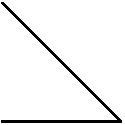### 2ex-2

#### 2.1source code in ex-2.rkt

 #lang racket (require (planet wcy/mpost-wrapper)) (provide main) (define (A-B-C) (values (point 0 0) (point '1cm 0) (point 0 '1cm))) (define (main) (draw (call-with-values A-B-C (lambda (A B C) (op-- A B C 'cycle)))))

#### 2.2the result of ex-2

Examples:

 > (require "examples/ex-2.rkt") > (make-figure "ex-2" main)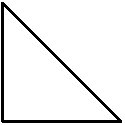### 3ex-3

#### 3.1source code in ex-3.rkt

 #lang racket (require (planet wcy/mpost-wrapper)) (provide main) (define (A-B-C-D) (values (point 0 0) (point '1cm 0) (point '1cm '1cm) (point 0 '1cm))) (define (main) (call-with-values A-B-C-D (lambda (A B C D) (draw (op-- A B C D 'cycle)) (draw (op-- A C)) (draw (op-- B D)))))

#### 3.2the result of ex-3

Examples:

 > (require "examples/ex-3.rkt") > (make-figure "ex-3" main)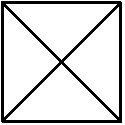### 4ex-4

#### 4.1source code in ex-4.rkt

 #lang racket (require (planet wcy/mpost-wrapper)) (provide main) (define (main) (draw (point 0 0) #:withpen (scale '4bp 'pencircle)))

#### 4.2the result of ex-4

Examples:

 > (require "examples/ex-4.rkt") > (make-figure "ex-4" main)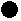### 5ex-5

#### 5.1source code in ex-5.rkt

 #lang racket (require (planet wcy/mpost-wrapper)) (provide main) (define (A-B-C) (values (point 0 0) (point '1cm 0) (point 0 '1cm))) (define (main) (call-with-values A-B-C (lambda (A B C) (draw (op-- A B C 'cycle)) (for ((p (in-list (list A B C)))) (draw p #:withpen (scale '4bp 'pencircle))) )))

#### 5.2the result of ex-5

Examples:

 > (require "examples/ex-5.rkt") > (make-figure "ex-5" main)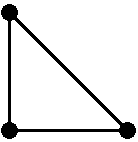### 6ex-6

#### 6.1source code in ex-6.rkt

 #lang racket (require (planet wcy/mpost-wrapper)) (provide main) (define (A-B-C-D) (values (point 0 0) (point '1cm 0) (point '1cm '1cm) (point 0 '1cm))) (define (main) (call-with-values A-B-C-D (lambda (A B C D) (draw (op-- A B C D 'cycle)) (draw (op-- A C)) (draw (op-- B D)) (for ((p (in-list (list A B C D)))) (draw p #:withpen (scale '4bp 'pencircle))))))

#### 6.2the result of ex-6

Examples:

 > (require "examples/ex-6.rkt") > (make-figure "ex-6" main)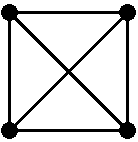### 7ex-7

#### 7.1source code in ex-7.rkt

 #lang racket (require (planet wcy/mpost-wrapper)) (provide main) (define (A-B-C) (values (point 0 0) (point '1cm 0) (point 0 '1cm))) (define (main) (call-with-values A-B-C (lambda (A B C) (draw (op-- A B  C 'cycle)) (draw (op-- (mediation '.5 B C) A)) (draw (op-- (mediation '.5 A C) B)) (draw (op-- (mediation '.5 B A) C)) )))

#### 7.2the result of ex-7

Examples:

 > (require "examples/ex-7.rkt") > (make-figure "ex-7" main)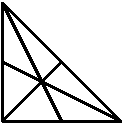### 8ex-8

#### 8.1source code in ex-8.rkt

 #lang racket (require (planet wcy/mpost-wrapper)) (provide main) (define (A-B-C) (values (point 0 0) (point '1cm 0) (point 0 '1cm))) (define (main) (call-with-values A-B-C (lambda (A B C) (draw (op-- A  B  C 'cycle)) (draw (op-- (mediation 0.5 B C) A)) (draw (op-- (mediation 0.5 A C) B)) (draw (op-- (mediation 0.5 B A) C)) (draw (op* 1/3  (op+ A B C)) #:withpen (scale '4bp 'pencircle)) )))

#### 8.2the result of ex-8

Examples:

 > (require "examples/ex-8.rkt") > (make-figure "ex-8" main)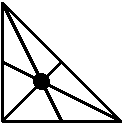### 9ex-9

#### 9.1source code in ex-9.rkt

 #lang racket (require (planet wcy/mpost-wrapper)) (provide main) (define (A-B-C) (values (point 0 0) (point '1cm 0) (point 0 '1cm))) (define (main) (call-with-values A-B-C (lambda (A B C) (draw (op-- A B C 'cycle)) (draw (op-- A B) #:withpen (scale '4bp 'pencircle)) )))

#### 9.2the result of ex-9

Examples:

 > (require "examples/ex-9.rkt") > (make-figure "ex-9" main)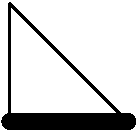### 10ex-10

#### 10.1source code in ex-10.rkt

 #lang racket (require (planet wcy/mpost-wrapper)) (provide main) (define (A-B-C) (values (point 0 0) (point '1cm 0) (point 0 '1cm))) (define (main) (call-with-values A-B-C (lambda (A B C) (draw (op-- A B C 'cycle)) (draw (op-- A B) #:withcolor (op+ 'green 'red)) )))

#### 10.2the result of ex-10

Examples:

 > (require "examples/ex-10.rkt") > (make-figure "ex-10" main)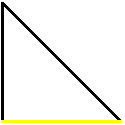### 11ex-11

#### 11.1source code in ex-11.rkt

 #lang racket (require (planet wcy/mpost-wrapper)) (provide main) (define (A-B-C) (values (point 0 0) (point '1cm 0) (point 0 '1cm))) (define (main) (call-with-values A-B-C (lambda (A B C) (draw (op-- A B)) (draw (op-- B C) #:dashed 'evenly) (draw (op-- C A) #:dashed 'withdots) )))

#### 11.2the result of ex-11

Examples:

 > (require "examples/ex-11.rkt") > (make-figure "ex-11" main)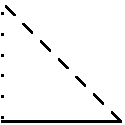### 12ex-12

#### 12.1source code in ex-12.rkt

 #lang racket (require (planet wcy/mpost-wrapper)) (provide main) (define (A-B-C) (values (point 0 0) (point '1cm 0) (point 0 '1cm))) (define (main) (call-with-values A-B-C (lambda (A B C) (for ((p (in-list (list (op-- A B) (op-- B C) (op-- C A) ))) (color (in-list (list 0.8 0.6 0.4)))) (draw p #:withpen (scale '2bp 'pencircle) #:withcolor (op* color 'white))) )))

#### 12.2the result of ex-12

Examples:

 > (require "examples/ex-12.rkt") > (make-figure "ex-12" main)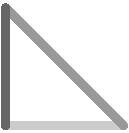### 13ex-13

#### 13.1source code in ex-13.rkt

 #lang racket (require (planet wcy/mpost-wrapper)) (provide main) (define (A-B-C-D) (values (point 0 0) (point '1cm 0) (point '1cm '1cm) (point 0 '1cm))) (define (main) (call-with-values A-B-C-D (lambda (A B C D) (draw (op-- A  B  C 'cycle)) (draw (op-- (mediation '.5 B C) A) #:dashed 'evenly) (draw (op-- (mediation '.5 A C) B) #:dashed 'evenly) (draw (op-- (mediation '.5 B A) C) #:dashed 'evenly) (draw (op* 1/3 (op+ A B C)) #:withpen (scale '4bp 'pencircle) #:withcolor 'red) )))

#### 13.2the result of ex-13

Examples:

 > (require "examples/ex-13.rkt") > (make-figure "ex-13" main)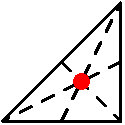### 14ex-14

#### 14.1source code in ex-14.rkt

 #lang racket (require (planet wcy/mpost-wrapper)) (provide main) (define (main) (draw (op-- (point 0 0) (point '3cm 0)) #:dashed (scale 2 'evenly)))

#### 14.2the result of ex-14

Examples:

 > (require "examples/ex-14.rkt") > (make-figure "ex-14" main)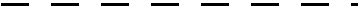### 15ex-15

#### 15.1source code in ex-15.rkt

 #lang racket (require (planet wcy/mpost-wrapper)) (provide main) (define (main) (draw (op-- (point 0 0) (point '3cm 0)) #:dashed (dashpattern "on 2bp off 3bp")))

#### 15.2the result of ex-15

Examples:

 > (require "examples/ex-15.rkt") > (make-figure "ex-15" main)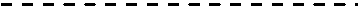### 16ex-16

#### 16.1source code in ex-16.rkt

 #lang racket (require (planet wcy/mpost-wrapper)) (provide main) (define (main) (draw (op-- (point 0 0) (point '3cm 0)) #:dashed (dashpattern "on 1bp off 2bp on 10bp off 2bp")))

#### 16.2the result of ex-16

Examples:

 > (require "examples/ex-16.rkt") > (make-figure "ex-16" main)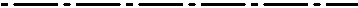### 17ex-17

#### 17.1source code in ex-17.rkt

 #lang racket (require (planet wcy/mpost-wrapper)) (provide main) (define (A-B-C) (values (point 0 0) (point '1cm 0) (point 0 '1cm))) (define (main) (call-with-values A-B-C (lambda (A B C) (drawarrow (op-- C B A)) (drawarrow (op-- A C) #:withpen (scale '2bp 'pencircle)))))

#### 17.2the result of ex-17

Examples:

 > (require "examples/ex-17.rkt") > (make-figure "ex-17" main)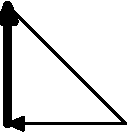### 18ex-18

#### 18.1source code in ex-18.rkt

 #lang racket (require (planet wcy/mpost-wrapper)) (provide main) (define (A-B-C) (values (point 0 0) (point '1cm 0) (point 0 '1cm))) (define (main) (call-with-values A-B-C (lambda (A B C) (drawdblarrow (op-- C B A 'cycle)) (drawdblarrow (op-- A C) #:withpen (scale '2bp 'pencircle)))))

#### 18.2the result of ex-18

Examples:

 > (require "examples/ex-18.rkt") > (make-figure "ex-18" main)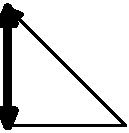### 19ex-19

#### 19.1source code in ex-19.rkt

 #lang racket (require (planet wcy/mpost-wrapper)) (provide main) (define (main) (draw (op-- (point '-1.5cm 0) (point '1.5cm 0))))

#### 19.2the result of ex-19

Examples:

 > (require "examples/ex-19.rkt") > (make-figure "ex-19" main)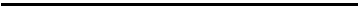### 20ex-20

#### 20.1source code in ex-20.rkt

 #lang racket (require (planet wcy/mpost-wrapper)) (provide main) (define (A-B-C) (values (point 0 0) (point '1cm 0) (point 0 '1cm))) (define (main) (call-with-values A-B-C (lambda (A B C) (fill (op-- A B C 'cycle) #:withcolor (op* 0.8 'white)))))

#### 20.2the result of ex-20

Examples:

 > (require "examples/ex-20.rkt") > (make-figure "ex-20" main)### 21ex-21

#### 21.1source code in ex-21.rkt

 #lang racket (require (planet wcy/mpost-wrapper)) (provide main) (define (A-B-C) (values (point 0 0) (point '1cm 0) (point 0 '1cm))) (define (main) (call-with-values A-B-C (lambda (A B C) (fill (op-- A B C 'cycle) #:withcolor (op* 0.8 'white)) (draw (op-- A B C 'cycle)))))

#### 21.2the result of ex-21

Examples:

 > (require "examples/ex-21.rkt") > (make-figure "ex-21" main)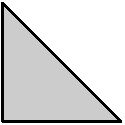### 22ex-22

#### 22.1source code in ex-22.rkt

 #lang racket (require (planet wcy/mpost-wrapper)) (provide main) (define (A-B-C) (values (point 0 0) (point '1cm 0) (point 0 '1cm))) (define (main) (call-with-values A-B-C (lambda (A B C) (fill (op-- A B C 'cycle) #:withcolor (op* 0.8 'white)) (draw (op-- A B C 'cycle) #:withpen (scale '2bp 'pencircle)))))

#### 22.2the result of ex-22

Examples:

 > (require "examples/ex-22.rkt") > (make-figure "ex-22" main)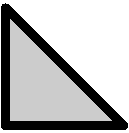### 23ex-23

#### 23.1source code in ex-23.rkt

 #lang racket (require (planet wcy/mpost-wrapper)) (provide main) (define (A-B-C) (values (point 0 0) (point '1cm 0) (point 0 '1cm))) (define (main) (call-with-values A-B-C (lambda (A B C) (draw (op-- A B C 'cycle) #:withpen (scale '2bp 'pencircle)) (fill (op-- A B C 'cycle) #:withcolor (op* 0.8 'white)))))

#### 23.2the result of ex-23

Examples:

 > (require "examples/ex-23.rkt") > (make-figure "ex-23" main)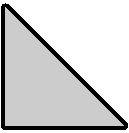### 24ex-24

#### 24.1source code in ex-24.rkt

 #lang racket (require (planet wcy/mpost-wrapper)) (provide main) (define (A-B-C-D) (values (point 0 0) (point '1cm 0) (point '1cm '1cm) (point 0 '1cm))) (define (main) (call-with-values A-B-C-D (lambda (A B C D) (fill (op--  A C B D 'cycle) #:withcolor (op* 0.8 'white)))))

#### 24.2the result of ex-24

Examples:

 > (require "examples/ex-24.rkt") > (make-figure "ex-24" main)### 25ex-25

#### 25.1source code in ex-25.rkt

 #lang racket (require (planet wcy/mpost-wrapper)) (provide main) (define (A-B-C-D) (values (point 0 0) (point '1cm 0) (point '1cm '1cm) (point 0 '1cm))) (define (main) (call-with-values A-B-C-D (lambda (A B C D) (let ((p (op--  A C B D 'cycle))) (fill p #:withcolor (op* 0.8 'white)) (draw p)))))

#### 25.2the result of ex-25

Examples:

 > (require "examples/ex-25.rkt") > (make-figure "ex-25" main)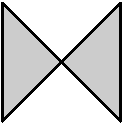### 26ex-26

#### 26.1source code in ex-26.rkt

 #lang racket (require (planet wcy/mpost-wrapper)) (provide main) (define (main) (let ((A (point '-1.5cm '-1.5cm)) (B (point '1.5cm '-1.5cm)) (C (point '1.5cm '1.5cm)) (D (point '-1.5cm '1.5cm))) (fill (op-- A C B D  'cycle) #:withcolor (op* 0.8 'white)) (draw (op-- (point '-1.5cm 0) (point '1.5cm '0))) (draw (op-- (point 0 '-1.5cm) (point 0 '1.5cm))) (drawarrow (op-- (point 0 0) (point '1cm 0)) #:withpen (scale '2bp 'pencircle)) (drawarrow (op-- (point 0 0) (point 0 '1cm)) #:withpen (scale '2bp 'pencircle))))

#### 26.2the result of ex-26

Examples:

 > (require "examples/ex-26.rkt") > (make-figure "ex-26" main)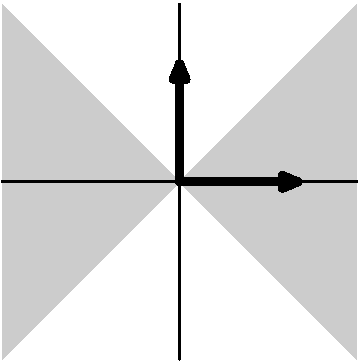### 27ex-27

#### 27.1source code in ex-27.rkt

 #lang racket (require (planet wcy/mpost-wrapper)) (require (planet wcy/mpost-wrapper/label)) (provide main) (define (main) (let ((A (point 0 0))) (draw A #:withpen (scale '4bp 'pencircle)) (label-top (btex "Au dessus") A) (label-bot  (btex "En dessous") A) (label-rt (btex "^^c0 droite") A) (label-lft  (btex "^^c0 gauche") A)))

#### 27.2the result of ex-27

Examples:

 > (require "examples/ex-27.rkt") > (make-figure "ex-27" main)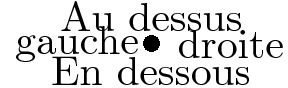### 28ex-28

#### 28.1source code in ex-28.rkt

 #lang racket (require (planet wcy/mpost-wrapper)) (require (planet wcy/mpost-wrapper/label)) (provide main) (define (main) (let ((A (point 0 0))) (draw A #:withpen (scale '4bp 'pencircle)) (label-ulft (btex "En haut ^^e0 gauche") A) (label-urt  (btex "En haut ^^e0 droite") A) (label-llft (btex "En bas ^^e0 gauche") A) (label-lrt  (btex "En bas ^^e0 droite") A)))

#### 28.2the result of ex-28

Examples:

 > (require "examples/ex-28.rkt") > (make-figure "ex-28" main)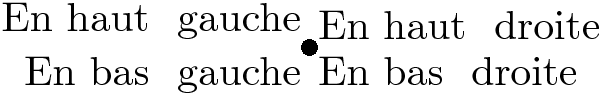### 29ex-29

#### 29.1source code in ex-29.rkt

 #lang racket (require (planet wcy/mpost-wrapper)) (require (planet wcy/mpost-wrapper/label)) (provide main) (define (main) (dot-label-urt (btex "$A$") (point 0 0)))

#### 29.2the result of ex-29

Examples:

 > (require "examples/ex-29.rkt") > (make-figure "ex-29" main)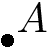### 30ex-30

#### 30.1source code in ex-30.rkt

 #lang racket (require (planet wcy/mpost-wrapper)) (require (planet wcy/mpost-wrapper/label)) (provide main) (define (A-B-C) (values (point 0 0) (point '1cm 0) (point 0 '1cm))) (define (main) (call-with-values A-B-C (lambda (A B C) (draw (op-- A B C 'cycle)) (dot-label-llft (btex "$A$") A) (dot-label-lrt (btex "$B$") B) (dot-label-top (btex "$C$") C))))

#### 30.2the result of ex-30

Examples:

 > (require "examples/ex-30.rkt") > (make-figure "ex-30" main)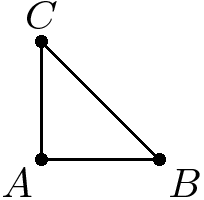### 31ex-31

#### 31.1source code in ex-31.rkt

 #lang racket (require (planet wcy/mpost-wrapper)) (require (planet wcy/mpost-wrapper/label)) (provide main) (define (A-B-C) (values (point 0 0) (point '1cm 0) (point 0 '1cm))) (define (main) (call-with-values A-B-C (lambda (A B C) (draw (op-- A B C 'cycle)) (label-bot (btex "$1$") (mediation  0.5 A B)) (label-lft (btex "$1$") (mediation  1/2 A C)) (label-urt (btex "$\\sqrt 2$") (mediation 1/2 B C)))))

#### 31.2the result of ex-31

Examples:

 > (require "examples/ex-31.rkt") > (make-figure "ex-31" main)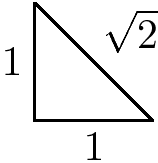### 32ex-32

#### 32.1source code in ex-32.rkt

 #lang racket (require (planet wcy/mpost-wrapper)) (require (planet wcy/mpost-wrapper/label)) (provide main) (define (ex-32-points y xs) (map(lambda (x) (point x y)) xs)) (define (main) (let* ((u '1cm) (xs (list  (op* -1 u) 0 u)) (u-points (ex-32-points u xs)) (l-points (ex-32-points 0 xs))) (for* ((a (in-list l-points)) (b (in-list u-points))) (draw  (op--  a b))) (for ((ut (in-list (list "$a$" "$b$" "$c$"))) (a  (in-list u-points)) (lt (in-list (list "$a'$" "$b'$" "$c'$"))) (b  (in-list l-points))) (dot-label-top (btex ut) a) (dot-label-bot (btex lt) b))))

#### 32.2the result of ex-32

Examples:

 > (require "examples/ex-32.rkt") > (make-figure "ex-32" main)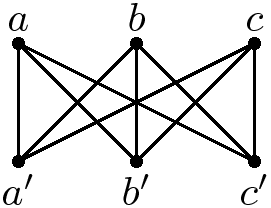### 33ex-33

#### 33.1source code in ex-33.rkt

 #lang racket (require (planet wcy/mpost-wrapper)) (provide main) (define (main) (draw (fullcircle)))

#### 33.2the result of ex-33

Examples:

 > (require "examples/ex-33.rkt") > (make-figure "ex-33" main)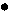### 34ex-34

#### 34.1source code in ex-34.rkt

 #lang racket (require (planet wcy/mpost-wrapper)) (provide main) (define (main) (draw (point 0 0 ) #:withpen (scale '4bp 'pencircle)))

#### 34.2the result of ex-34

Examples:

 > (require "examples/ex-34.rkt") > (make-figure "ex-34" main)### 35ex-35

#### 35.1source code in ex-35.rkt

 #lang racket (require (planet wcy/mpost-wrapper)) (provide main) (define (main) (draw (point 0 0 ) #:withpen (scale '4bp 'pencircle)))

#### 35.2the result of ex-35

Examples:

 > (require "examples/ex-35.rkt") > (make-figure "ex-35" main)### 36ex-36

#### 36.1source code in ex-36.rkt

 #lang racket (require (planet wcy/mpost-wrapper)) (provide main) (define (A-B-C) (values (point 0 0) (point '1cm 0) (point 0 '1cm))) (define (main) (call-with-values A-B-C (lambda (A B C) (draw (op-- A B C 'cycle)) (for ((a-point (in-list (list A B C)))) (fill (fullcircle  '4bp a-point) #:withcolor 'white)) (for ((a-point (in-list (list A B C)))) (draw  (fullcircle  '4bp a-point))))))

#### 36.2the result of ex-36

Examples:

 > (require "examples/ex-36.rkt") > (make-figure "ex-36" main)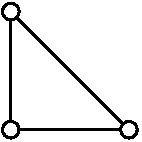### 37ex-37

#### 37.1source code in ex-37.rkt

 #lang racket (require (planet wcy/mpost-wrapper)) (provide main) (define (A-B-C-D) (values (point 0 0) (point '1cm 0) (point '1cm '1cm) (point 0 '1cm))) (define (main) (call-with-values A-B-C-D (lambda (A B C D) (draw (op.. A D B C)) (for ((A (in-list (list A B C D)))) (draw A #:withpen (scale '4bp 'pencircle))))))

#### 37.2the result of ex-37

Examples:

 > (require "examples/ex-37.rkt") > (make-figure "ex-37" main)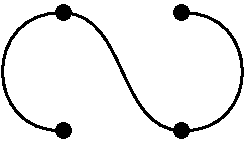### 38ex-38

#### 38.1source code in ex-38.rkt

 #lang racket (require (planet wcy/mpost-wrapper)) (provide main) (define (A-B-C-D) (values (point 0 0) (point '1cm 0) (point '1cm '1cm) (point 0 '1cm))) (define (main) (call-with-values A-B-C-D (lambda (A B C D) (draw (path A '-- D '..  B '.. C)) )))

#### 38.2the result of ex-38

Examples:

 > (require "examples/ex-38.rkt") > (make-figure "ex-38" main)### 39ex-39

#### 39.1source code in ex-39.rkt

 #lang racket (require (planet wcy/mpost-wrapper)) (provide main) (define (A-B-C-D) (values (point 0 0) (point '1cm 0) (point '1cm '1cm) (point 0 '1cm))) (define (main) (call-with-values A-B-C-D (lambda (A B C D) (draw (path A '--- D '..  B '.. C)) )))

#### 39.2the result of ex-39

Examples:

 > (require "examples/ex-39.rkt") > (make-figure "ex-39" main)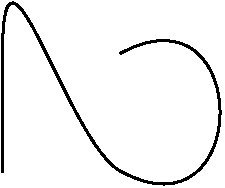### 40ex-40

#### 40.1source code in ex-40.rkt

 #lang racket (require (planet wcy/mpost-wrapper)) (provide main) (define (A-B-C-D) (values (point 0 0) (point '1cm 0) (point '1cm '1cm) (point 0 '1cm))) (define (main) (call-with-values A-B-C-D (lambda (A B C D) (draw (path A '..  D '.. B '.. C)) )))

#### 40.2the result of ex-40

Examples:

 > (require "examples/ex-40.rkt") > (make-figure "ex-40" main)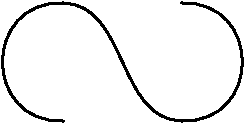### 41ex-41

#### 41.1source code in ex-41.rkt

 #lang racket (require (planet wcy/mpost-wrapper)) (provide main) (define (A-B-C-D) (values (point 0 0) (point '1cm 0) (point '1cm '1cm) (point 0 '1cm))) (define (main) (call-with-values A-B-C-D (lambda (A B C D) (draw (op.. A B C D 'cycle) ))))

#### 41.2the result of ex-41

Examples:

 > (require "examples/ex-41.rkt") > (make-figure "ex-41" main)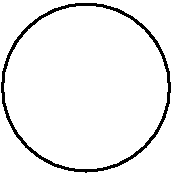### 42ex-42

#### 42.1source code in ex-42.rkt

 #lang racket (require (planet wcy/mpost-wrapper)) (provide main) (define (A-B-C-D) (values (point 0 0) (point '1cm 0) (point '1cm '1cm) (point 0 '1cm))) (define (main) (call-with-values A-B-C-D (lambda (A B C D) (draw (op.. A  C  'cycle) ))))

#### 42.2the result of ex-42

Examples:

 > (require "examples/ex-42.rkt") > (make-figure "ex-42" main)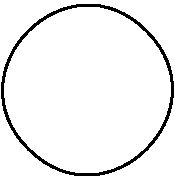### 43ex-43

#### 43.1source code in ex-43.rkt

 #lang racket (require (planet wcy/mpost-wrapper)) (provide main) (define (main) (draw (path (point 0    0) (dir-spec (point 0 1))  ".." (point '2cm 0) (dir-spec (point 1 0)))))

#### 43.2the result of ex-43

Examples:

 > (require "examples/ex-43.rkt") > (make-figure "ex-43" main)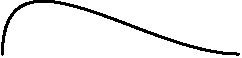### 44ex-44

#### 44.1source code in ex-44.rkt

 #lang racket (require (planet wcy/mpost-wrapper)) (provide main) (define (main) (draw (path (point 0    0) (dir-spec (dir 90))  ".." (point '2cm 0) (dir-spec (dir 0)))))

#### 44.2the result of ex-44

Examples:

 > (require "examples/ex-44.rkt") > (make-figure "ex-44" main)### 45ex-45

#### 45.1source code in ex-45.rkt

 #lang racket (require (planet wcy/mpost-wrapper)) (provide main) (define (main) (draw (path (point 0    0) (dir-spec 'up)  ".." (point '2cm 0) (dir-spec 'right))))

#### 45.2the result of ex-45

Examples:

 > (require "examples/ex-45.rkt") > (make-figure "ex-45" main)### 46ex-46

#### 46.1source code in ex-46.rkt

 #lang racket (require (planet wcy/mpost-wrapper)) (provide main) (define (main) (draw (path (point 0    0) (dir-spec 'up)  ".." (point '2cm 0) (dir-spec 'up))))

#### 46.2the result of ex-46

Examples:

 > (require "examples/ex-46.rkt") > (make-figure "ex-46" main)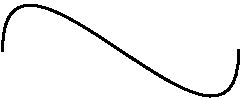### 47ex-47

#### 47.1source code in ex-47.rkt

 #lang racket (require (planet wcy/mpost-wrapper)) (provide main) (define (main) (draw (path (point 0    0) (dir-spec 'up)  ".." (point '2cm 0) (dir-spec 'up)  ".." 'cycle)))

#### 47.2the result of ex-47

Examples:

 > (require "examples/ex-47.rkt") > (make-figure "ex-47" main)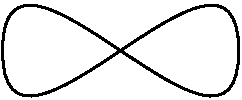### 48ex-48

#### 48.1source code in ex-48.rkt

 #lang racket (require (planet wcy/mpost-wrapper)) (provide main) (define (arc O A B rou) (let ((alpha (op-angle (op- A O))) (beta  (op-angle (op- B O)))) (path (op+ O (polar rou alpha)) (dir-spec (dir (op+ alpha 90))) ".." (op+ O (polar rou beta )) (dir-spec (dir (op+ beta  90)))))) (define (main) (let* ((O (point 0 0)) (alpha 30) (A (polar '2cm 0)) (B (polar '2cm alpha))) (draw (path O ".." A)) (draw (path O ".." B)) (draw (arc O A B '1cm))))

#### 48.2the result of ex-48

Examples:

 > (require "examples/ex-48.rkt") > (make-figure "ex-48" main)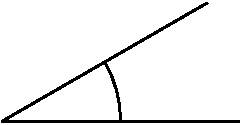### 49ex-49

#### 49.1source code in ex-49.rkt

 #lang racket (require (planet wcy/mpost-wrapper)) (provide main) (define (arc O A B rou) (let ((alpha (op-angle (op- A O))) (beta  (op-angle (op- B O)))) (path (op+ O (polar rou alpha)) (dir-spec (dir (op+ alpha 90))) ".." (op+ O (polar rou beta )) (dir-spec (dir (op+ beta  90)))))) (define (main) (let* ((O (point 0 0)) (alpha 30) (A (polar '2cm 0)) (B (polar '2cm alpha))) (draw (path O ".." A)) (draw (path O ".." B)) (draw (arc O A B '1cm)) (draw (arc O A B '1.1cm))))

#### 49.2the result of ex-49

Examples:

 > (require "examples/ex-49.rkt") > (make-figure "ex-49" main)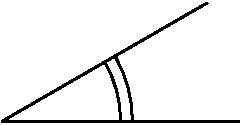### 50ex-50

#### 50.1source code in ex-50.rkt

 #lang racket (require (planet wcy/mpost-wrapper)) (provide main) (define (arc O A B rou) (let ((alpha (op-angle (op- A O))) (beta  (op-angle (op- B O)))) (path (op+ O (polar rou alpha)) (dir-spec (dir (op+ alpha 90))) ".." (op+ O (polar rou beta )) (dir-spec (dir (op+ beta  90)))))) (define (draw-angle A B C n) (let ((rou '4.5mm)) (if (> n 0) (begin (draw (arc A B C (op+ rou (op* n '0.5mm)))) (draw-angle  A B C (- n 1))) (void)))) (define (main) (let ((A (point 0 0)) (B (point '3cm 0)) (C (point '1cm '2cm))) (draw (op-- A B C 'cycle)) (draw-angle A B C 1) (draw-angle B C A 2) (draw-angle C A B 3)))

#### 50.2the result of ex-50

Examples:

 > (require "examples/ex-50.rkt") > (make-figure "ex-50" main)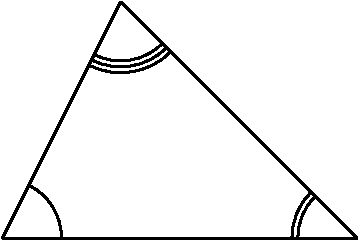### 51ex-51

#### 51.1source code in ex-51.rkt

 #lang racket (require (planet wcy/mpost-wrapper)) (provide main) (define (main) (let* ((u '1cm) (p (path (point u 0) (dir-spec 'up)  ".." (point (op* -1 u) 0) (dir-spec 'down) "--" 'cycle))) (fill p #:withcolor (op* 0.8 'white)) (draw p #:withpen (scale '1bp 'pencircle)) ))

#### 51.2the result of ex-51

Examples:

 > (require "examples/ex-51.rkt") > (make-figure "ex-51" main)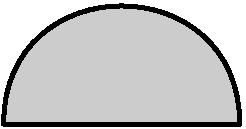### 52ex-52

#### 52.1source code in ex-52.rkt

 #lang racket (require (planet wcy/mpost-wrapper)) (provide main) (define (main) (let* ((u '1cm) (p (path (point u 0)            (dir-spec 'up) ".." (point (op* -1   u) 0) (dir-spec 'down) "--" (point (op* -0.2 u) 0) (dir-spec 'up) ".." (point (op*  0.2 u) 0) (dir-spec 'down) "--" 'cycle))) (fill p #:withcolor (op* 0.8 'white)) (draw p #:withpen (scale '1bp 'pencircle)) ))

#### 52.2the result of ex-52

Examples:

 > (require "examples/ex-52.rkt") > (make-figure "ex-52" main)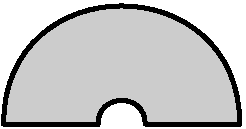### 53ex-53

#### 53.1source code in ex-53.rkt

 #lang racket (require (planet wcy/mpost-wrapper)) (provide main) (define (main) (let* ((u '1cm) (A  (point (op*  0.2 u) (op*  0.05 u))) (AA (point (op*  0.2 u) (op* -0.05 u))) (B  (point (op*  1.0 u) (op*  0.05 u))) (BB (point (op*  1.0 u) (op* -0.05 u))) (C  (point (op* -0.2 u) (op*  0    0))) (D  (point (op* -1.0 u) (op*  0    0))) (p (path B  (dir-spec 'up) ".." D  (dir-spec 'down) ".." BB (dir-spec 'up) "--" AA (dir-spec 'down) ".." C  (dir-spec 'up) ".." A  (dir-spec 'down) "--" 'cycle))) (fill p #:withcolor (op* 0.8 'white)) (draw p #:withpen (scale '1bp 'pencircle)) ))

#### 53.2the result of ex-53

Examples:

 > (require "examples/ex-53.rkt") > (make-figure "ex-53" main)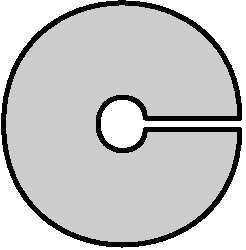### 54ex-54

#### 54.1source code in ex-54.rkt

 #lang racket (require (planet wcy/mpost-wrapper)) (provide main) (define (main) (let* ((u '1cm) (A (point 0 0)) (B (polar u  60)) (C (polar u -60)) (D (point (op* 2 u) 0))) (for ((x (in-list (list A B C D)))) (draw x #:withpen (scale '4bp 'pencircle))) (draw (op-- A D B D C)) (for ((x (in-list (list (path A (dir-spec 'up) ".." B) (path A (dir-spec 'down) ".." C) (path A (dir-spec (dir  30)) ".." B) (path A (dir-spec (dir -30)) ".." C))))) (draw x) )))

#### 54.2the result of ex-54

Examples:

 > (require "examples/ex-54.rkt") > (make-figure "ex-54" main)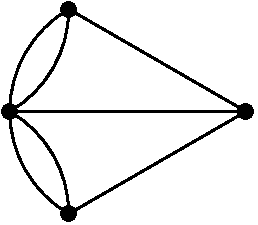### 55ex-55

#### 55.1source code in ex-55.rkt

 #lang racket (require (planet wcy/mpost-wrapper)) (provide main) (define (main) (draw (op.. (point 0 0)  (point '1cm '1cm) (point '2cm 0))))

#### 55.2the result of ex-55

Examples:

 > (require "examples/ex-55.rkt") > (make-figure "ex-55" main)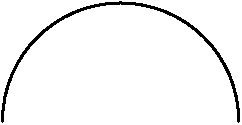### 56ex-56

#### 56.1source code in ex-56.rkt

 #lang racket (require (planet wcy/mpost-wrapper)) (provide main) (define (main) (draw (path (point 0 0) '.. "tension 2" '.. (point '1cm '1cm) '.. (point '2cm 0))))

#### 56.2the result of ex-56

Examples:

 > (require "examples/ex-56.rkt") > (make-figure "ex-56" main)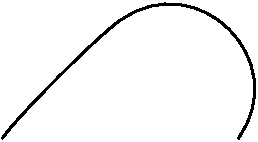### 57ex-57

#### 57.1source code in ex-57.rkt

 #lang racket (require (planet wcy/mpost-wrapper)) (provide main) (define (main) (let ((u '1cm)) (draw (path (polar u  90) (dir-spec (dir 0))    '.. (polar u  -150) (dir-spec (dir 120))  '.. (polar u  -30 ) (dir-spec (dir -120))  '.. 'cycle))))

#### 57.2the result of ex-57

Examples:

 > (require "examples/ex-57.rkt") > (make-figure "ex-57" main)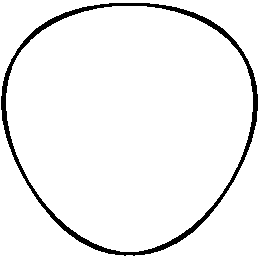### 58ex-58

#### 58.1source code in ex-58.rkt

 #lang racket (require (planet wcy/mpost-wrapper)) (provide main) (define (main) (let ((u '1cm) (tension " tension 2 ")) (draw (path (polar u  90) (dir-spec (dir 0))    '.. tension '.. (polar u  -150 ) (dir-spec (dir 120))  '.. tension '.. (polar u  -30 ) (dir-spec (dir -120))  '.. tension '.. 'cycle))))

#### 58.2the result of ex-58

Examples:

 > (require "examples/ex-58.rkt") > (make-figure "ex-58" main)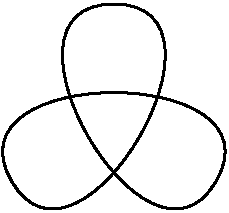### 59ex-59

#### 59.1source code in ex-59.rkt

 #lang racket (require (planet wcy/mpost-wrapper)) (provide main) (define (main) (let ((u '1cm) (tension " tension 4 ")) (draw (path (polar u  90) (dir-spec (dir 0))    '.. tension '.. (polar u  -150 ) (dir-spec (dir 120))  '.. tension '.. (polar u  -30 ) (dir-spec (dir -120))  '.. tension '.. 'cycle))))

#### 59.2the result of ex-59

Examples:

 > (require "examples/ex-59.rkt") > (make-figure "ex-59" main)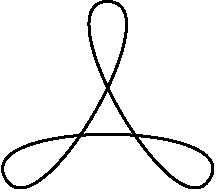### 60ex-60

#### 60.1source code in ex-60.rkt

 #lang racket (require (planet wcy/mpost-wrapper)) (provide main) (define (main) (let* ((u '0.5cm) (A (point 0 0)) (B (point (op* -1 u) (op* 2 u))) (C (point (op* 4  u) (op* 3 u))) (D (point (op* 3  u) 0))) (draw (path A ".." " controls " B " and " C ".." D) #:withpen (scale '2pt 'pencircle)) (draw (op-- B C) #:dashed 'evenly) (drawarrow (op-- A B)) (drawarrow (op-- D C))))

#### 60.2the result of ex-60

Examples:

 > (require "examples/ex-60.rkt") > (make-figure "ex-60" main)### 61ex-61

#### 61.1source code in ex-61.rkt

 #lang racket (require (planet wcy/mpost-wrapper)) (provide main) (define (main) (define (f z1 zn) (if (null? zn) z1 (let ((x (f2 z1 zn))) (f (car x) (cdr x))))) (define (f2 z1 zn) (if (null? (cdr zn)) (list (mediation 1/2 z1 (car zn))) (cons (mediation 1/2 z1 (car zn)) (f2 (car zn) (cdr zn))))) (let* ((u '1cm) (A (point 0 0)) (B (point (op* -1 u) (op* 2 u))) (C (point (op* 3  u) (op* 3 u))) (D (point (op* 2  u) 0))) (for ((A (in-list (list A B C D)))) (draw A #:withpen (scale '4bp 'pencircle))) (draw (path A ".." " controls " B " and " C ".." D)) (draw (f A (list B C D)) #:withpen (scale '4bp 'pencircle))))

#### 61.2the result of ex-61

Examples:

 > (require "examples/ex-61.rkt") > (make-figure "ex-61" main)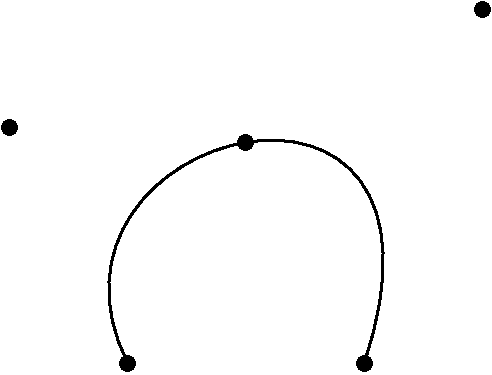### 62ex-62

#### 62.1source code in ex-62.rkt

 #lang racket (require (planet wcy/mpost-wrapper)) (provide main) (define (main) (define  (bezier n A B C D) (let* ((a1 A) (a2 B) (a3 C) (a4 D) (a12 (mediation 1/2 a1   a2)) (a23 (mediation 1/2 a2   a3)) (a34 (mediation 1/2 a3   a4)) (a123 (mediation 1/2  a12  a23)) (a234 (mediation 1/2  a23  a34)) (a1234(mediation 1/2  a123 a234))) (if (> n 0) (begin (bezier (- n 1) a1 a12 a123 a1234) (bezier (- n 1) a1234 a234 a34 a4)) (draw a1234 #:withpen (scale '2bp 'pencircle))))) (let* ((u '1cm) (A (point 0 0)) (B (point (op* -1 u) (op* 2 u))) (C (point (op* 3  u) (op* 3 u))) (D (point (op* 2  u) 0))) (for ((A (in-list (list A B C D)))) (draw A #:withpen (scale '4bp 'pencircle))) ;; (draw (path A ".." " controls " B " and " C ".." D)) (bezier 5 A B C D)))

#### 62.2the result of ex-62

Examples:

 > (require "examples/ex-62.rkt") > (make-figure "ex-62" main)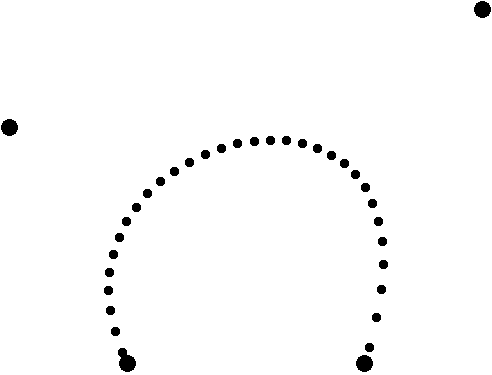### 63ex-63

#### 63.1source code in ex-63.rkt

 #lang racket (require (planet wcy/mpost-wrapper)) (provide main) (define (main) (draw (path (point 0 0) (dir-spec 'up) '.. (point '1cm '1mm) ".." (point '2cm 0) (dir-spec 'down))))

#### 63.2the result of ex-63

Examples:

 > (require "examples/ex-63.rkt") > (make-figure "ex-63" main)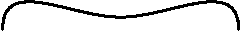### 64ex-64

#### 64.1source code in ex-64.rkt

 #lang racket (require (planet wcy/mpost-wrapper)) (provide main) (define (main) (draw (path (point 0 0) (dir-spec 'up) '... (point '1cm '1mm) "..." (point '2cm 0) (dir-spec 'down))))

#### 64.2the result of ex-64

Examples:

 > (require "examples/ex-64.rkt") > (make-figure "ex-64" main)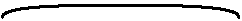### 65ex-65

#### 65.1source code in ex-65.rkt

 #lang racket (require (planet wcy/mpost-wrapper)) (provide main) (define (A-B-C-D) (values (point 0 0) (point '1cm 0) (point '1cm '1cm) (point 0 '1cm))) (define (main) (call-with-values A-B-C-D (lambda (A B C D) (draw (path A " {curl 0} " ".." D ".." B ".." C )))))

#### 65.2the result of ex-65

Examples:

 > (require "examples/ex-65.rkt") > (make-figure "ex-65" main)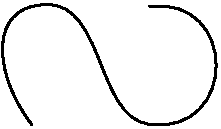### 66ex-66

#### 66.1source code in ex-66.rkt

 #lang racket (require (planet wcy/mpost-wrapper)) (provide main) (define (A-B-C-D) (values (point 0 0) (point '1cm 0) (point '1cm '1cm) (point 0 '1cm))) (define (main) (call-with-values A-B-C-D (lambda (A B C D) (draw (path A " {curl 1} " ".." D ".." B ".." C )))))

#### 66.2the result of ex-66

Examples:

 > (require "examples/ex-66.rkt") > (make-figure "ex-66" main)### 67ex-67

#### 67.1source code in ex-67.rkt

 #lang racket (require (planet wcy/mpost-wrapper)) (provide main) (define (A-B-C-D) (values (point 0 0) (point '1cm 0) (point '1cm '1cm) (point 0 '1cm))) (define (main) (call-with-values A-B-C-D (lambda (A B C D) (draw (path A " {curl 2} " ".." D ".." B ".." C )))))

#### 67.2the result of ex-67

Examples:

 > (require "examples/ex-67.rkt") > (make-figure "ex-67" main)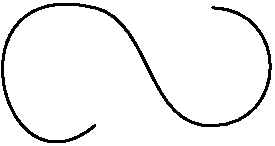### 68ex-68

#### 68.1source code in ex-68.rkt

 #lang racket (require (planet wcy/mpost-wrapper)) (provide main) (define (main) (let ((A (unknown-point)) (B (unknown-point)) (C (unknown-point)) (D (unknown-point))) (eqn= (xpart A) (ypart A) (ypart B) (xpart C) 0) (eqn= (xpart B) (ypart C) (xpart D) (ypart D) '2cm) (draw (op-- A B C D 'cycle) #:withpen (scale '2bp 'pencircle))))

#### 68.2the result of ex-68

Examples:

 > (require "examples/ex-68.rkt") > (make-figure "ex-68" main)### 69ex-69

#### 69.1source code in ex-69.rkt

 #lang racket (require (planet wcy/mpost-wrapper)) (provide main) (define (main) (let* ((A (point 0 0)) (B (point '2cm '3cm)) (C (point '1cm 0)) (D (point '-1cm '2cm)) (M (unknown-point)) (lambda1 (unknown-numeric)) (mu (unknown-numeric))) (eqn= M  (mediation lambda1  A B) (mediation mu C D)) (draw (op-- A B)) (draw (op-- C D)) (draw M #:withpen (scale '4bp 'pencircle))))

#### 69.2the result of ex-69

Examples:

 > (require "examples/ex-69.rkt") > (make-figure "ex-69" main)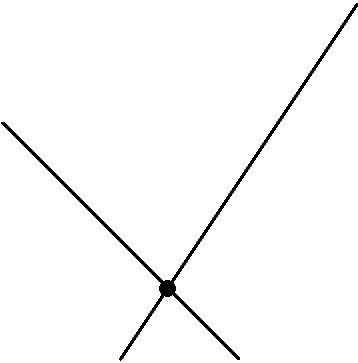### 70ex-70

#### 70.1source code in ex-70.rkt

 #lang racket (require (planet wcy/mpost-wrapper)) (provide main) (define (main) (let* ((A (point 0 0)) (B (point '2cm '3cm)) (C (point '1cm 0)) (D (point '-1cm '2cm)) (M (unknown-point))) (eqn= M  (mediation (whatever)  A B) (mediation (whatever) C D)) (draw (op-- A B)) (draw (op-- C D)) (draw M #:withpen (scale '4bp 'pencircle))))

#### 70.2the result of ex-70

Examples:

 > (require "examples/ex-70.rkt") > (make-figure "ex-70" main)### 71ex-71

#### 71.1source code in ex-71.rkt

 #lang racket (require (planet wcy/mpost-wrapper)) (provide main) (define (main) (let* ((A (point 0 0)) (B (point '3cm 0)) (C (point '1cm '2cm)) (AA (mediation 0.5 B C)) (BB (mediation 0.5 A C)) (CC (mediation 0.5 A B)) (G (unknown-point))) (eqn= G (mediation (whatever) A AA) (mediation (whatever) B BB)) (draw (op-- A B C 'cycle)) (for ((a (list A B C)) (b (list AA BB CC))) (draw  (op-- a b) #:dashed  'withdots)) (draw G #:withpen (scale '4bp 'pencircle))))

#### 71.2the result of ex-71

Examples:

 > (require "examples/ex-71.rkt") > (make-figure "ex-71" main)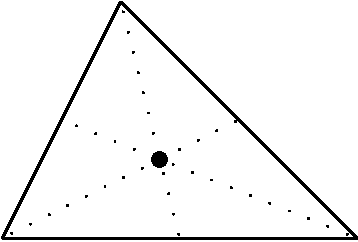### 72ex-72

#### 72.1source code in ex-72.rkt

 #lang racket (require (planet wcy/mpost-wrapper)) (provide main) (define (main) (let ((p  (fullcircle '5mm)) (x (point '1mm '2mm))) (for ((n (in-range 5))) (draw (shift  (op* n x) p )))))

#### 72.2the result of ex-72

Examples:

 > (require "examples/ex-72.rkt") > (make-figure "ex-72" main)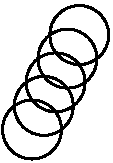### 73ex-73

#### 73.1source code in ex-73.rkt

 #lang racket (require (planet wcy/mpost-wrapper)) (provide main) (define (main) (let ((p (path (point '5mm '-5mm) (dir-spec 'right) '.. (point '2cm 0)))) (for ((n (in-range 10))) (draw (rotate (* 10 n) p)))))

#### 73.2the result of ex-73

Examples:

 > (require "examples/ex-73.rkt") > (make-figure "ex-73" main)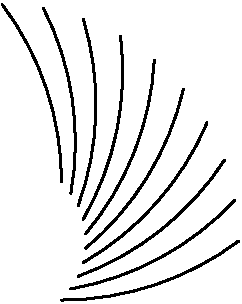### 74ex-74

#### 74.1source code in ex-74.rkt

 #lang racket (require (planet wcy/mpost-wrapper)) (provide main) (define (main) (let ((p  (fullcircle '5mm)) (x (point '1mm '2mm))) (for ((n (in-range 1 5))) (draw (xscale n p)))))

#### 74.2the result of ex-74

Examples:

 > (require "examples/ex-74.rkt") > (make-figure "ex-74" main)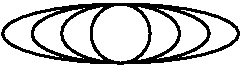### 75ex-75

#### 75.1source code in ex-75.rkt

 #lang racket (require (planet wcy/mpost-wrapper)) (provide main) (define (main) (let* ((A (point '1cm 0)) (B (rotate 72 A)) (C (rotate 72 B)) (D (rotate 72 C)) (E (rotate 72 D))) (draw (op-- A B C D E 'cycle))))

#### 75.2the result of ex-75

Examples:

 > (require "examples/ex-75.rkt") > (make-figure "ex-75" main)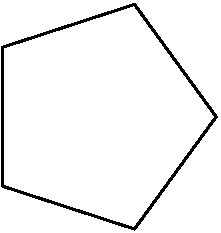### 76ex-76

#### 76.1source code in ex-76.rkt

 #lang racket (require (planet wcy/mpost-wrapper)) (provide main) (define (main) (let* ((A (point '1cm 0)) (B (rotate 72 A)) (C (rotate 72 B)) (D (rotate 72 C)) (E (rotate 72 D))) (draw (op-- A C  E B D 'cycle))))

#### 76.2the result of ex-76

Examples:

 > (require "examples/ex-76.rkt") > (make-figure "ex-76" main)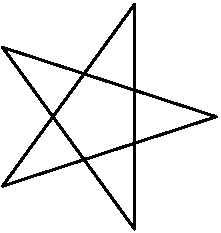### 77ex-77

#### 77.1source code in ex-77.rkt

 #lang racket (require (planet wcy/mpost-wrapper)) (provide main) (define (main) (let* ((A (point '1cm 0)) (B (rotate 72 A)) (C (rotate 72 B)) (D (rotate 72 C)) (E (rotate 72 D)) (AA (unknown-point)) (BB (unknown-point)) (CC (unknown-point)) (DD (unknown-point)) (EE (unknown-point))) (eqn= AA (mediation (whatever) A C)) (eqn= AA (mediation (whatever) B D)) (eqn= BB (mediation (whatever) B D)) (eqn= BB (mediation (whatever) C E)) (eqn= CC (mediation (whatever) C E)) (eqn= CC (mediation (whatever) D A)) (eqn= DD (mediation (whatever) D A)) (eqn= DD (mediation (whatever) E B)) (eqn= EE (mediation (whatever) E B)) (eqn= EE (mediation (whatever) A C)) (fill (op-- A C  E B D 'cycle) #:withcolor (op* 0.8 'white)) (fill (op-- AA  BB  CC  DD  EE  'cycle) #:withcolor 'white) (draw (op-- A  C  E  B  D  'cycle))))

#### 77.2the result of ex-77

Examples:

 > (require "examples/ex-77.rkt") > (make-figure "ex-77" main)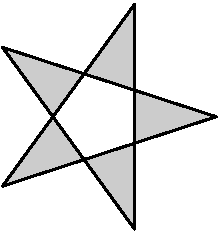### 78ex-78

#### 78.1source code in ex-78.rkt

 #lang racket (require (planet wcy/mpost-wrapper)) (provide main) (define (main) (let ((p (path (op-- (point 0 0) (point '1cm 0))))) (drawarrow p #:withpen (scale '2bp 'pencircle)) (drawarrow (zscale (point 1 2) p))))

#### 78.2the result of ex-78

Examples:

 > (require "examples/ex-78.rkt") > (make-figure "ex-78" main)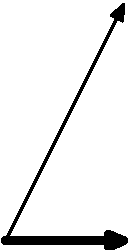### 79ex-79

#### 79.1source code in ex-79.rkt

 #lang racket (require (planet wcy/mpost-wrapper)) (provide main) (define (main) (let* ((u '1cm) (p (path (op-- (point 0 0) (point u 0)))) (A (point u (op* -1 u))) (alpha 90)) (drawarrow p #:withpen (scale '1bp 'pencircle)) (drawarrow ((rotate-around A alpha) p)) (drawarrow (shift A (rotate alpha (shift (op* -1 A) p))) #:withpen (scale '1bp 'pencircle) #:dashed 'withdots)))

#### 79.2the result of ex-79

Examples:

 > (require "examples/ex-79.rkt") > (make-figure "ex-79" main)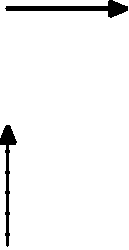### 80ex-80

#### 80.1source code in ex-80.rkt

 #lang racket (require (planet wcy/mpost-wrapper)) (require (planet wcy/mpost-wrapper/label)) (provide main) (define (main) (let* ((p (path (point 0 0) '-- (point '1cm 0))) (A (point '1cm '-1cm)) (alpha 90)) (drawarrow p #:withpen (scale '1bp 'pencircle)) (draw A #:withpen (scale '4bp 'pencircle)) (label-ulft (btex "$A$") A) (draw (point 0 0) #:withpen (scale '4bp 'pencircle)) (label-bot (btex "$O$") (point 0 0)) (let loop ((i 0)) (if (< i 10) (begin (drawarrow (shift  (op* -1 (op/ (op* i A) 10)) p) #:withcolor 'red) (drawarrow (rotate (op/ (op* i alpha) 10) (shift (op* -1 A) p)) #:withcolor 'green) (drawarrow (shift  (op/ (op* i A) 10) (rotate alpha (shift (op* -1 A) p))) #:withcolor 'blue) (loop (+ 1 i))) (void)))))

#### 80.2the result of ex-80

Examples:

 > (require "examples/ex-80.rkt") > (make-figure "ex-80" main)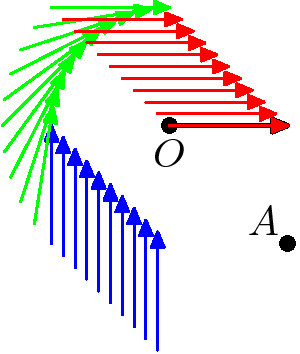### 81ex-81

#### 81.1source code in ex-81.rkt

 #lang racket (require (planet wcy/mpost-wrapper)) (provide main) (define (main) (define (f AA A B C) (eqn= (op- AA A) (rotate  90 (op* (unknown-numeric) (op- B C)))) (eqn= AA (mediation (whatever) B C))) (let* ((A (point 0 0))